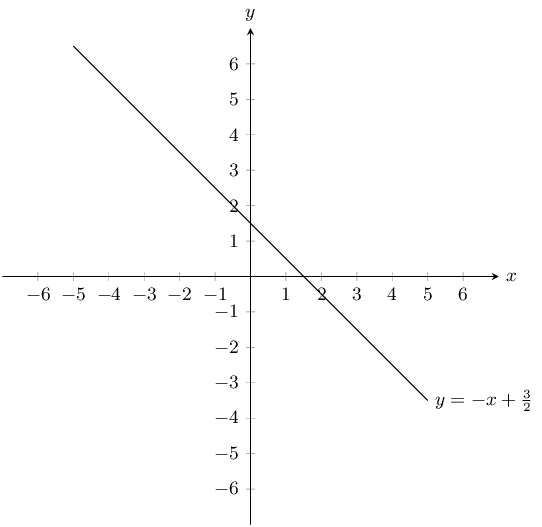Home Practice
For learners and parents For teachers and schools
Textbooks
Full catalogue
Pricing SupportLog in

We think you are located in United States. Is this correct?

# End of chapter exercises

Textbook Exercise 6.8

Complete the following tables and identify the function.

$\begin{array}{| l | l | l | l | l | l | l |} \hline x & & 2 & 3 & 4 & & 6 \\ \hline y & 3 & 6 & & 12 & 15 & \\ \hline \end{array}$
$\begin{array}{| l | l | l | l | l | l | l |} \hline x & 1 & 2 & 3 & 4 & 5 & 6 \\ \hline y & 3 & 6 & 9 & 12 & 15 & 18 \\ \hline \end{array}$ $y = 3x$
$\begin{array}{| l | l | l | l | l | l | l |} \hline x & 1 & & & 4 & 5 & 6 \\ \hline y & -3 & -2 & -1 & & 1 & 2 \\ \hline \end{array}$
$\begin{array}{| l | l | l | l | l | l | l |} \hline x & 1 & 2 & 3 & 4 & 5 & 6 \\ \hline y & -3 & -2 & -1 & 0 & 1 & 2 \\ \hline \end{array}$ $y = x -4$

Plot the following points on a graph.

$\begin{array}{| l | l | l | l | l | l | l |} \hline x & 1 & 2 & 3 & 4 & 5 & 6 \\ \hline y & 1 & 2 & 3 & 4 & 5 & 6 \\ \hline \end{array}$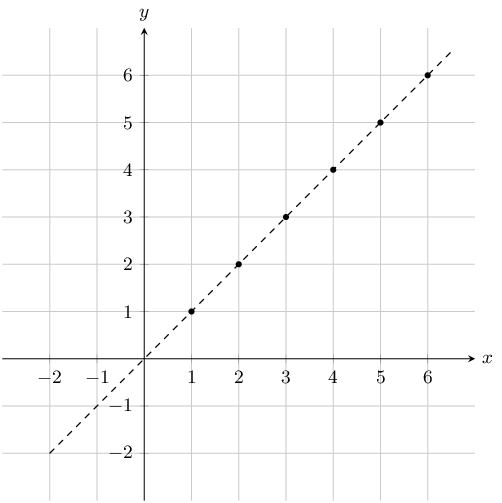$\begin{array}{| l | l | l | l | l | l | l |} \hline x & 50 & 100 & 150 & 200 & 250 & 300 \\ \hline y & 1 & 2 & 3 & 4 & 5 & 6 \\ \hline \end{array}$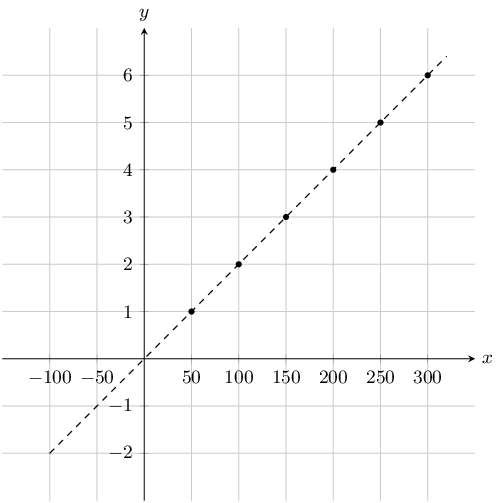Create a table of values from the function given and then plot the function. Your table must have at least 5 ordered pairs.

$$x^2 - 4$$
$\begin{array}{| l | l | l | l | l | l | l | l |} \hline x & -3 & -2 & -1 & 0 & 1 & 2 & 3 \\ \hline y & 5 & 0 & -3 & -4 & -3 & 0 & 5 \\ \hline \end{array}$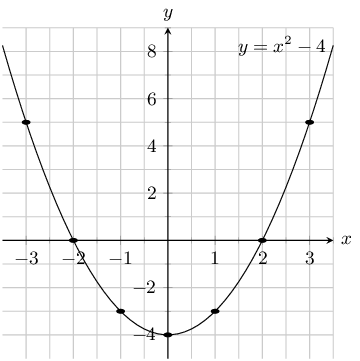$$y = 4x - 1$$
$\begin{array}{| l | l | l | l | l | l |} \hline x & -1 & -\text{0,5} & 0 & \text{0,5} & 1 \\ \hline y & -5 & -3 & -1 & 1 & 3 \\ \hline \end{array}$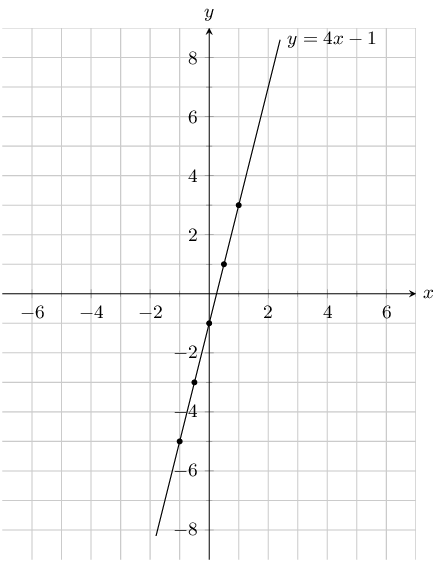Determine the $$y$$-intercept and the $$x$$-intercepts of the function.

$$y = - 3 x - 5$$

\begin{align*} y & = - 3 x - 5 \\ y & = - 3 (0) - 5 \\ y & = -5 \\ \therefore c &= -5 \end{align*} \begin{align*} y & = - 3 x - 5 \\ (0) & = - 3 x - 5 \\ 5 & = - 3 x \\ - \frac{5}{3} & = x \end{align*}

$$x$$-intercept $$=- \frac{5}{3}$$ and $$y$$-intercept $$=-5$$

$$y = 2 x + 4$$

\begin{align*} y & = 2 x + 4 \\ y & = 2 (0) + 4 \\ y & = 4 \\ \therefore c &= 4 \end{align*} \begin{align*} y & = 2 x + 4 \\ (0) & = 2 x + 4 \\ -4 & = 2 x \\ -2 & = x \end{align*}

$$x$$-intercept $$=-2$$ and $$y$$-intercept $$=4$$

The graph below shows an equation, which has the form $$y = mx + c$$. Calculate or otherwise find the values of $$m$$ (the gradient of the line) and $$c$$ (the $$y$$-intercept of the line).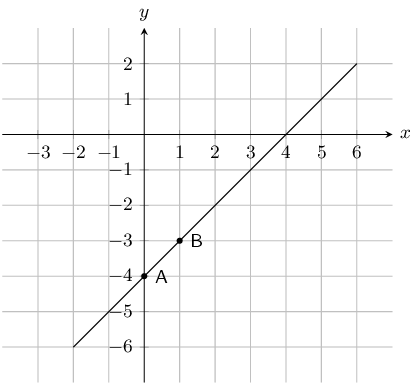Point $$A$$ is the $$y$$-intercept. Point $$A$$ has co-ordinates $$(0;-4)$$ and so $$c = -4$$.

\begin{align*} y & = mx + c \\ (-3) & = m(1) - 4 -3 & = m - 4 \\ 1 & = m \end{align*}

Therefore $$m = 1$$, and $$y = x -4$$.

Look at the graphs below. Each graph is labelled with a letter. In the questions that follow, match any given equation with the label of a corresponding graph.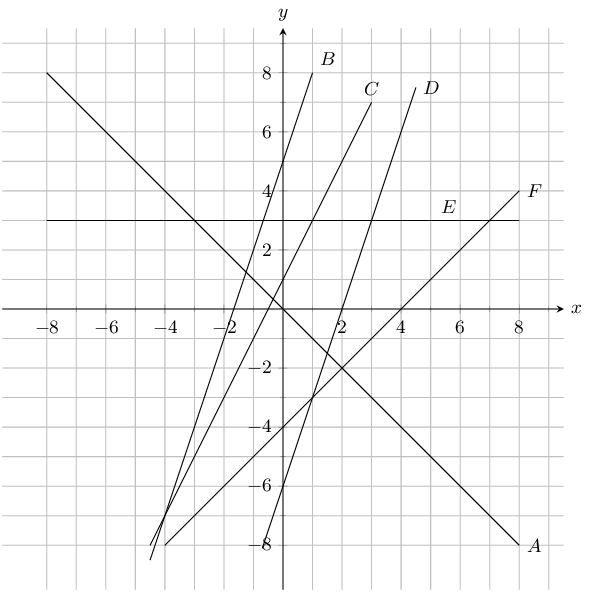$$y = 3$$
$$E$$
$$y = 3x + 5$$
$$B$$
$$y = -x$$
$$A$$
$$y = 2x + 1$$
$$C$$
$$y = x - 4$$
$$F$$
$$y = 3x - 6$$
$$D$$

State whether the following is true or not

The $$y$$-intercept of $$y + 5 = x$$ is $$-5$$.

True

The gradient of $$-y = x + 2$$ is $$1$$.

False

\begin{align*} -y &= x + 2 \\ y &= -x - 2 \end{align*}

The gradient of $$-4y = 3$$ is $$1$$.

False

\begin{align*} -4y &= 3 \\ y &= -\frac{3}{4} \end{align*}

Write the following in standard form:

$$2y - 5x = 6$$
\begin{align*} 2y - 5x &= 6 \\ 2y&= 5x + 6 \\ y & = \frac{5}{2}x + 3 \end{align*}
$$6y - 3x = 5x + 1$$
\begin{align*} 6y - 3x &= 5x + 1 \\ 6y&= 8x + 1 \\ y = & \frac{4}{3}x + \frac{1}{6} \end{align*}

Sketch the graphs of the following:

$$y=2x+4$$

The $$y$$-intercept is $$(0;4)$$ and the $$x$$-intercept is $$(-2;0)$$.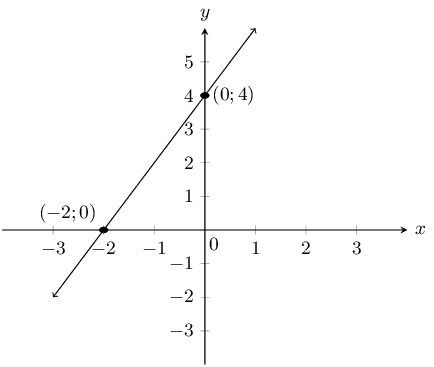$$y-3x=0$$

Write the equation in standard form: $$y = 3x$$.

The $$y$$-intercept is $$(0;0)$$ and the $$x$$-intercept is $$(0;0)$$.

We note the following pairs of values: $$(1;3)$$, $$(2;6)$$, $$(-1;-3)$$ and $$(-2;-6)$$. Now we can draw the graph.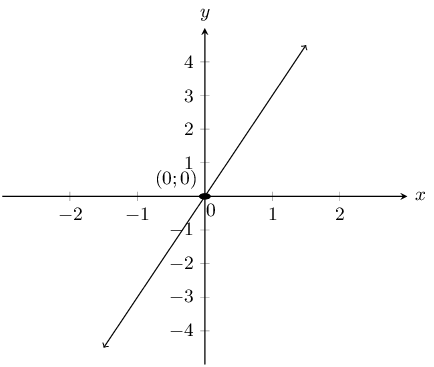$$2y=4-x$$

Write the equation in standard form: $$y = -\frac{1}{2}x + 2$$.

The $$y$$-intercept is $$(0;2)$$ and the $$x$$-intercept is $$(4;0)$$.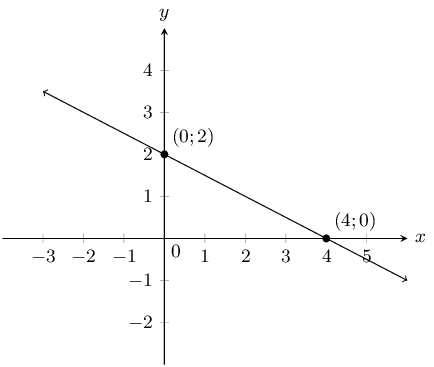The function for how much water a tap dispenses is given by: $$V = 60t$$, where $$x \text { and } V$$ are in seconds and $$\text{mL}$$ respectively.

Use this information to answer the following:

Evaluate $$V(2)$$.

\begin{align*} V(2) &= \text{60}(2) \\ &=\text{120}\text{ mL} \end{align*}

Evaluate $$V(10)$$.

\begin{align*} V(10) &= \text{60}(10) \\ &= \text{600}\text{ mL} \end{align*}

How long will it take to fill a $$\text{2}$$ $$\text{L}$$ bottle of water?

\begin{align*} V(t) &= \text{2 000}t \\ t &= \frac{\text{2 000}}{60} \\ t &= \text{33,33}s \end{align*}

How much water can the tap dispense in $$\text{4}$$ $$\text{s}$$?

\begin{align*} V(4) &= 60(4) \\ &= \text{240}\text{ mL} \end{align*}

The graph below shows the distance travelled by a car over time, where $$s(t)$$ is distance in $$\text{km}$$ and $$t$$ is time in minutes.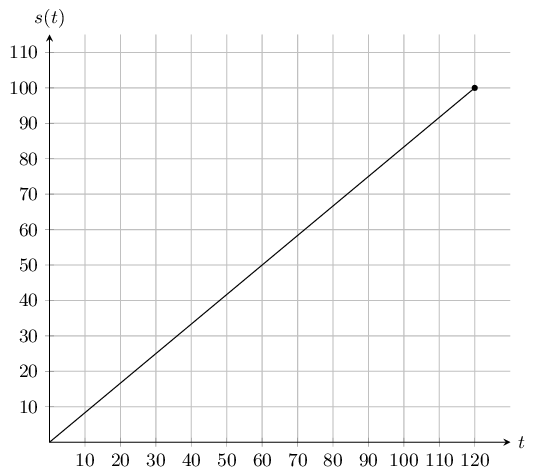Use this information to answer the following:

What distance did the car travel in an hour?

50km

What is the domain of the function?

The domain is $$0 \le t \le 120$$ min.

What is the range of the function? What does it represent?

The range is $$0 \le s \le 100$$ km, it represents the total distance travelled.

On the graph here you see a function of the form: $$y = ax^2 + q$$. Two points on the parabola are shown: Point A, the turning point of the parabola, at $$(\text{0};\text{6})$$, and Point B is at $$\left(\text{3}; 3\right)$$. Calculate the values of $$a$$ and $$q$$.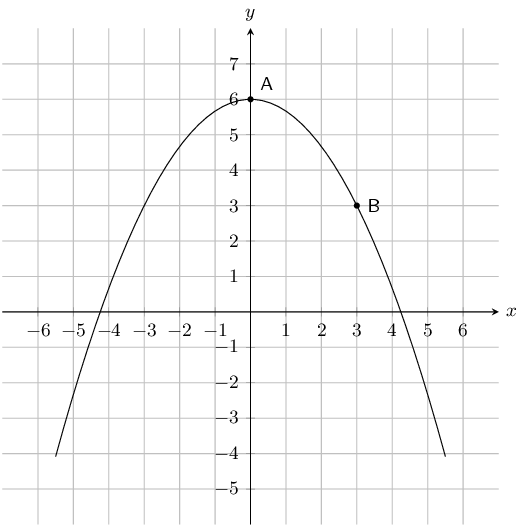The value of $$q$$ is 6.

\begin{align*} y & = ax^2 +6 \\ \left( 3 \right) & = a(\text{3})^2 +6 \quad \longleftarrow \quad ^{\text{substitute in the}}_{\text{coordinates of a point!}} \\ 3 & = \text{9}a +6 \\ 3 -6 & = \text{9}a \\ -3 & = \text{9}a \\ - \frac{1}{3} & = a \end{align*} $a = - \frac{1}{3};~ q = 6$

Given the following equation:

$$y= -5x^{2} + 3$$

Calculate the $$y$$-coordinate of the $$y$$-intercept.

\begin{align*} y & = ax^2 + q \\ & = - 5 x^{2} + 3 \\ & = - 5 (0)^{2} + 3\\ & = 0+3 \end{align*}

The $$y$$-coordinate of the $$y$$-intercept is $$3$$.

Now calculate the $$x$$-intercepts. Your answer must be correct to 2 decimal places.

\begin{align*} y & = - 5 x^{2} + 3 \\ (0) & =- 5 x^{2} + 3 \\ 5 x^{2} &=3\\ x^2 &= \frac{3}{5} \\ x & =\pm \sqrt{\frac{3}{5}}\\ \text{Therefore: } x = +\sqrt{\frac{3}{5}} &\text{ and } x = - \sqrt{\frac{3}{5}} \\ x= -\text{0,77} &\text{ and } x= \text{0,77} \end{align*}

The $$x$$-intercepts are $$(-\text{0,77};0)$$ and $$(\text{0,77};0)$$.

Given the following graph, identify a function that matches each of the given equations: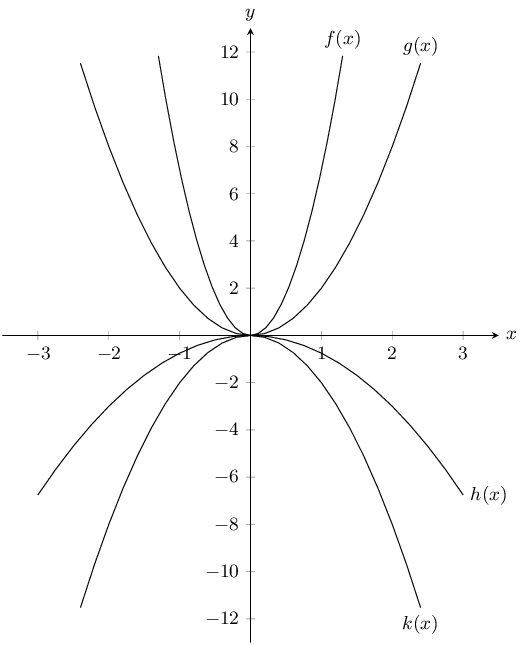$$y = -2x^2$$

$$k(x)$$

$$y = 2x^2$$

$$g(x)$$

$$y = -\text{0,75}x^2$$

$$h(x)$$

$$y = 7x^2$$

$$f(x)$$

Given the following graph, identify a function that matches each of the given equations: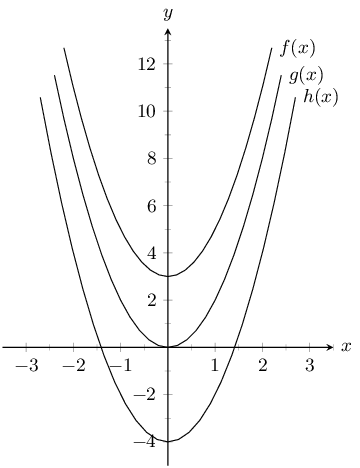$$y = 2x^2$$

$$g(x)$$

$$y = 2x^2 + 3$$

$$f(x)$$

$$y = 2x^2 - 4$$

$$h(x)$$

Sketch the following functions:

$$y=x^{2}+3$$

The $$y$$-intercept is $$(0;3)$$. There are no $$x$$-intercepts.

$$a$$ is positive and so the graph is a smile with a minimum turning point at $$(0;3)$$.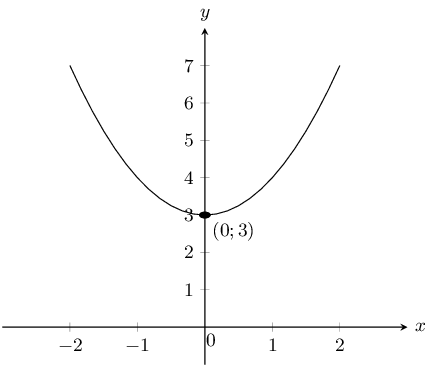$$y=\frac{1}{2}x^{2}+4$$

The $$y$$-intercept is $$(0;4)$$. There are no $$x$$-intercepts.

$$a$$ is positive and so the graph is a smile with a minimum turning point at $$(0;4)$$.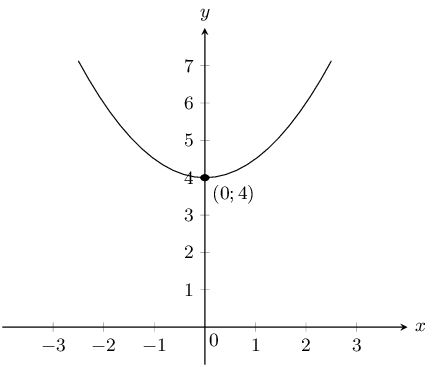$$y=2x^{2}-4$$

The $$y$$-intercept is $$(0;-4)$$. The $$x$$-intercepts are at $$\left(\sqrt{2};0\right)$$ and $$\left(-\sqrt{2};0\right)$$.

$$a$$ is positive and so the graph is a smile with a minimum turning point at $$(0;-4)$$.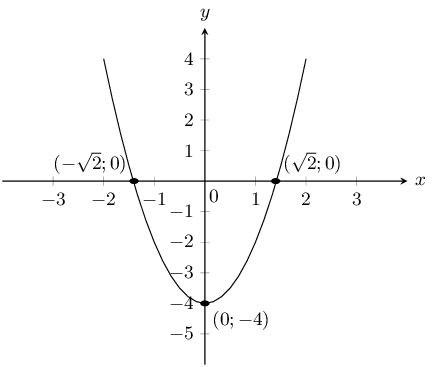Sebastian and Lucas dive into a pool from different heights. Their midair paths can be described by the following quadratic equations: $$y = -2x^2 +8$$ for Sebastian and $$y = -\frac{2}{3}x^2 + 6$$ for Lucas.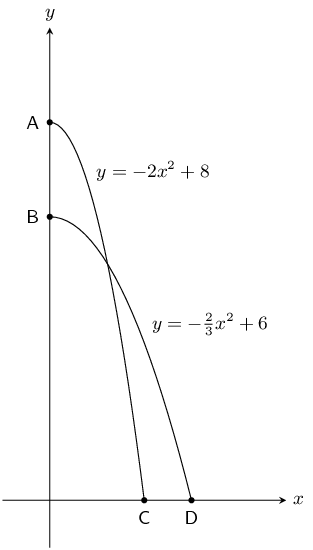From what height did Sebastian dive?

Maximum value of $$y = -2x^2 +8$$ is $$\text{8}$$ $$\text{m}$$

From what height did Lucas dive?

Maximum value of $$y = -\frac{2}{3}x^2 + 6$$ is $$\text{6}$$ $$\text{m}$$

How far from the pool wall did Lucas land?

\begin{align*} y = &-\frac{2}{3}x^2 + 6 \\ 0 = &-\frac{2}{3}x^2 + 6 \\ \frac{3}{2}x^2 - 6&= 0 \\ x^2 - 9 &= 0 \\ (x - 3)(x + 3) &= 0\\ \therefore & x = \text{3}\text{ m} \end{align*}

Lucas landed $$\text{3}$$ $$\text{m}$$ from the pool wall.

How much closer to the pool wall did Sebastian land compared to Lucas?

\begin{align*} y &= -2x^2 +8 \\ 0 &= -2x^2 +8 \\ 2x^2-8 &= 0 \\ x^2 - 4 &= 0 \\ (x - 2)(x + 2) &= 0\\ \therefore & x = \text{2}\text{ m} \end{align*}

Sebastian landed $$\text{2}$$ $$\text{m}$$ from the pool wall.

Therefore Sebastian landed $$\text{1}$$ $$\text{m}$$ closer to the wall than Lucas.

The following graph shows a hyperbolic equation of the form $$y = \dfrac {a}{x} + q$$. Point A is shown at $$\left( -1 ; -5 \right)$$. Calculate the values of $$a$$ and $$q$$.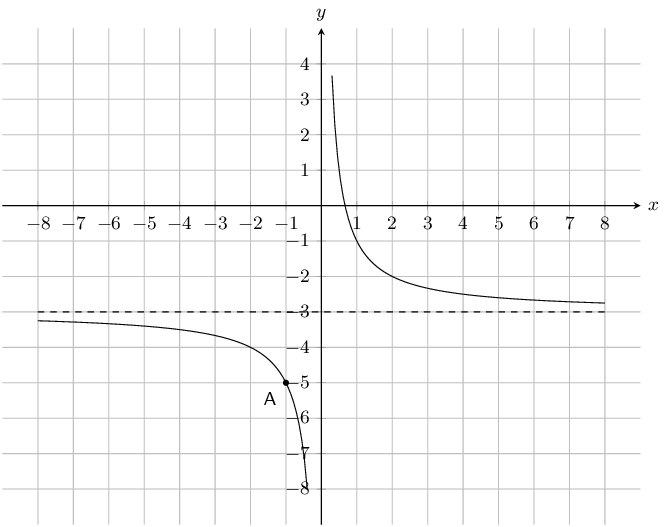\begin{align*} q & = -3 \\ \\ y & = \frac {a}{x} -3 \\ \left( -5 \right) & = \dfrac {a}{ \left( -1 \right) } -3 \end{align*} \begin{align*} -1 \left( -5 \right) & = \left[ \dfrac {a}{-1} -3 \right] \left( -1 \right) \\ \text{5} & = a + \text{3} \\ \text{2} & = a \end{align*}

Therefore $$a = 2$$ and $$q = -3$$.

The equation is $$y = \dfrac {2}{x} -3$$.

Given the following equation: $y = - \frac{3}{x} +4$

Determine the location of the $$y$$-intercept.

\begin{align*} y & = - \frac{3}{x} +4 \\ y & = - \frac{3}{(0)} +4\\ & \text{no solution} \end{align*}

There is no $$y$$-intercept.

Determine the location of the $$x$$-intercept.

\begin{align*} y & = - \frac{3}{x} +4 \\ (0) & = - \frac{3}{x} +4 \\ (x)(0) & = \left[ - \frac{3}{x} +4 \right](x) \\ 0 & = -3 +4x \\ 3 & = 4 x \\ x & = \frac{3}{4} \end{align*}

The $$x$$-intercept is at $$\left(\frac{3}{4};0\right)$$.

Given the following graph, identify a function that matches each of the given equations: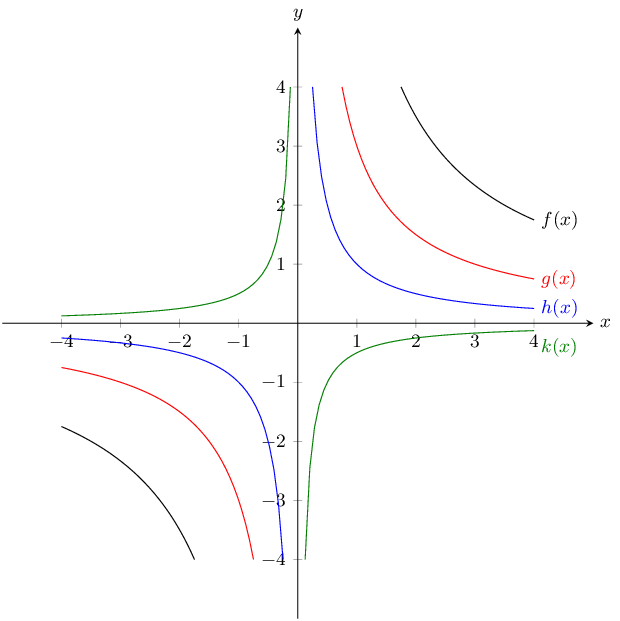$$y = -\dfrac{1}{2x}$$

$$k(x)$$

$$y = \dfrac{7}{x}$$

$$f(x)$$

$$y = \dfrac{3}{x}$$

$$g(x)$$

$$y = \dfrac{1}{x}$$

$$h(x)$$

Sketch the following functions and identify the asymptotes:

$$y=\dfrac{3}{x}+4$$

The asymptote is $$y = 4$$.

$$a$$ is positive and so the graph lies in the first and third quadrants.

There is no $$y$$-intercept. The $$x$$-intercept is at $$\left(-\frac{3}{4};0\right)$$.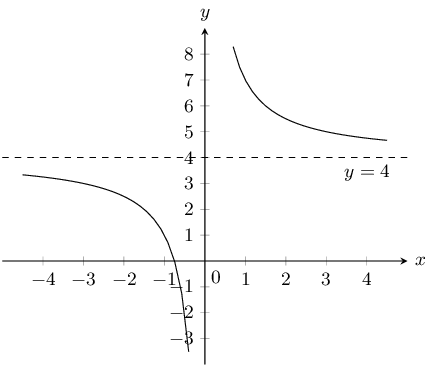$$y=\dfrac{1}{x}$$

The asymptote is $$y = 0$$.

$$a$$ is positive and so the graph lies in the first and third quadrants.

There is no $$y$$-intercept and no $$x$$-intercept.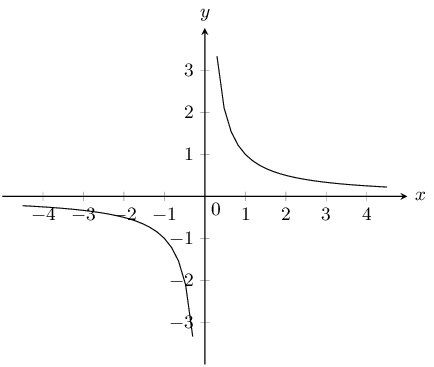$$y=\dfrac{2}{x} - 2$$

The asymptote is $$y = -2$$.

$$a$$ is positive and so the graph lies in the first and third quadrants.

There is no $$y$$-intercept. The $$x$$-intercept is at $$(1;0)$$.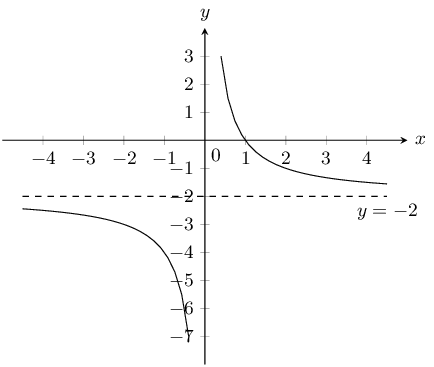Sketch the functions given and describe the transformation used to obtain the second function. Show all asymptotes.

$$y = \dfrac{2}{x}$$ and $$\dfrac{2}{x} + 2$$

$$y = \dfrac{2}{x}$$:

The asymptote is $$y = 0$$.

$$a$$ is positive and so the graph lies in the first and third quadrants.

There is no $$y$$-intercept and no $$x$$-intercept.

$$y = \dfrac{2}{x} + 2$$:

The asymptote is $$y = 2$$.

$$a$$ is positive and so the graph lies in the first and third quadrants.

There is no $$y$$-intercept. The $$x$$-intercept is at $$(-1;0)$$.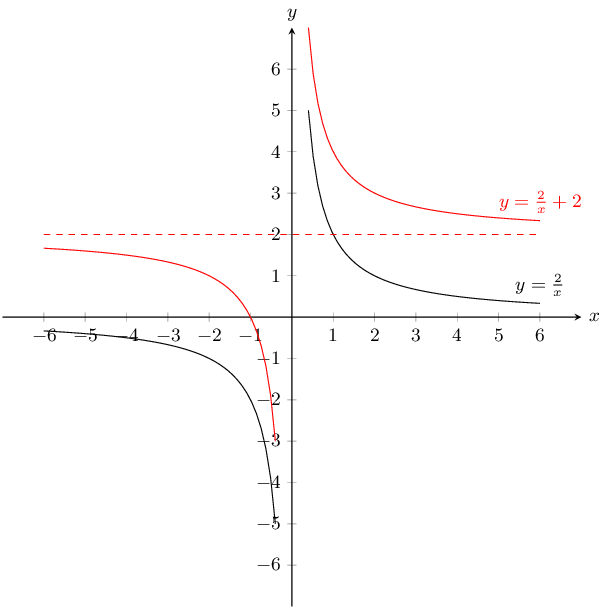Translation by 2 in the positive $$y$$-direction.

$$y = \dfrac{2}{x}$$ and $$\dfrac{1}{2x}$$

$$y = \dfrac{2}{x}$$:

The asymptote is $$y = 0$$.

$$a$$ is positive and so the graph lies in the first and third quadrants.

There is no $$y$$-intercept and no $$x$$-intercept.

$$y = \dfrac{1}{2x}$$:

The asymptote is $$y = 0$$.

$$a$$ is positive and so the graph lies in the first and third quadrants.

There is no $$y$$-intercept and no $$x$$-intercept.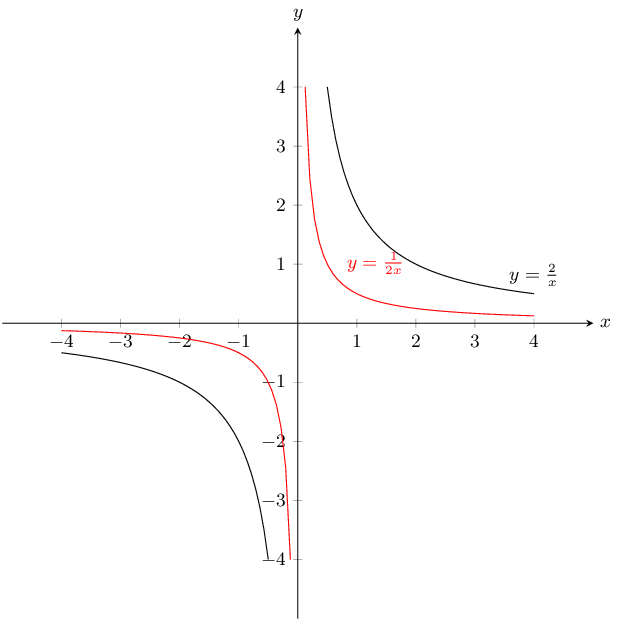Reduction by 4

$$y = \dfrac{3}{x}$$ and $$y = \dfrac{3x + 3}{x}$$

First simplify the second equation:

$$y = \dfrac{3x + 3}{x} = \dfrac{3}{x} + 1$$

$$y = \dfrac{3}{x}$$:

The asymptote is $$y = 0$$.

$$a$$ is positive and so the graph lies in the first and third quadrants.

There is no $$y$$-intercept and no $$x$$-intercept.

$$y = \dfrac{3}{x} + 1$$:

The asymptote is $$y = 1$$.

$$a$$ is positive and so the graph lies in the first and third quadrants.

There is no $$y$$-intercept. The $$x$$-intercept is at $$(-1;0)$$.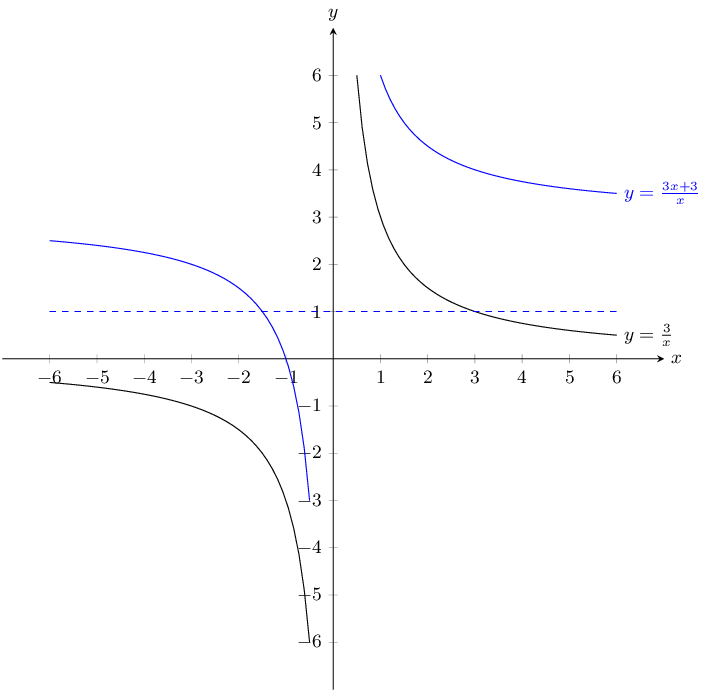Translation by 3 units in the positive $$y$$-direction.

$$y = \dfrac{3}{x}$$ and $$y = -\dfrac{3}{x}$$

$$y = \dfrac{3}{x}$$:

The asymptote is $$y = 0$$.

$$a$$ is positive and so the graph lies in the first and third quadrants.

There is no $$y$$-intercept and no $$x$$-intercept.

$$y = -\dfrac{3}{x}$$:

The asymptote is $$y = 0$$.

$$a$$ is negative and so the graph lies in the second and fourth quadrants.

There is no $$y$$-intercept and no $$x$$-intercept.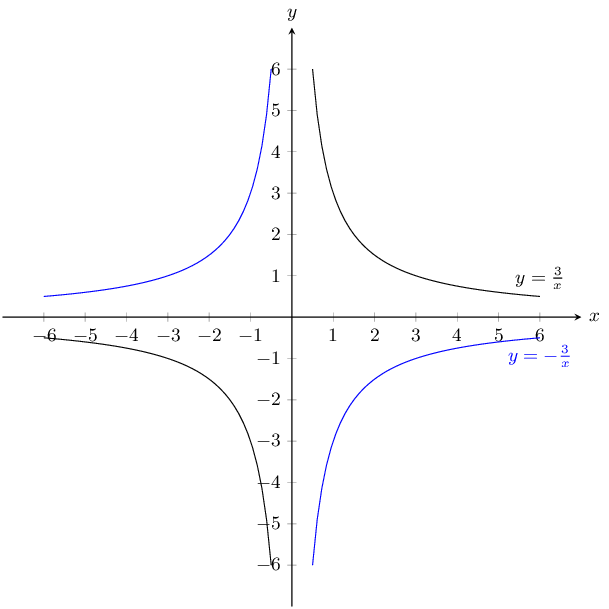Reflection on $$x$$-axis

Given the following equation:

$$y = -\frac{1}{2} \cdot (4)^{x} + 3$$

Calculate the $$y$$-intercept. Your answer must be correct to 2 decimal places.

\begin{align*} y & = \left( -\frac{1}{2} \right). (4)^{x} + 3 \\ & = \left( -\frac{1}{2} \right). (4)^{(0)} + 3 \\ & = \left( -\frac{1}{2} \right). (1) + 3 \\ & = (-\text{0,5}) + 3 \\ & = \text{2,50} \end{align*}

The $$y$$-intercept is $$(0;\text{2,50})$$.

Now calculate the $$x$$-intercept. Estimate your answer to one decimal place if necessary.

We calculate the $$x$$-intercept by letting $$y = 0$$. Then start to solve for $$x$$.

\begin{align*} 0 & = \left( -\frac{1}{2} \right) \cdot (4)^{x} + 3\\ -3 & = \left( -\frac{1}{2} \right)\cdot (4)^{x} \\ \left(-2\right)(-3) & = \left(-2\right) \left(-\frac{1}{2}\right)\cdot (4)^{x} \\ 6 & = 4^{x} \end{align*} \begin{align*} \text{Try: } 4^0 & = 1\\ \text{Try: } 4^1 & = 4\\ \text{Try: } 4^2 & = 16 \end{align*}

We can see that the exponent must be between 1 and 2. By trial and error we get $$\text{1,3}$$. Therefore the $$x$$-intercept is $$(\text{1,3};0)$$.

Sketch the following functions and identify the asymptotes:

$$y=3^{x}+2$$

The $$y$$-intercept is $$(0;2)$$. There is no $$x$$-intercept. The asymptote is at $$y = 2$$.

$$a > 1$$ therefore the graph curves upwards.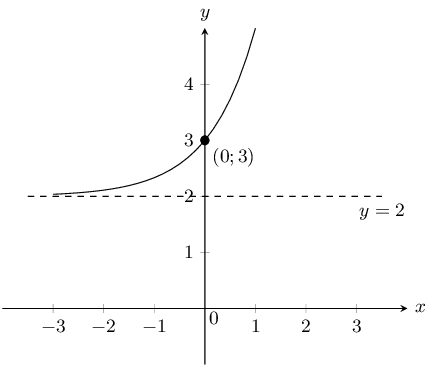$$y=-4\times 2^{x}$$

The $$y$$-intercept is $$(0;-4)$$. There is no $$x$$-intercept. The asymptote is at $$y = 0$$.

$$a < 1$$ therefore the graph curves downwards.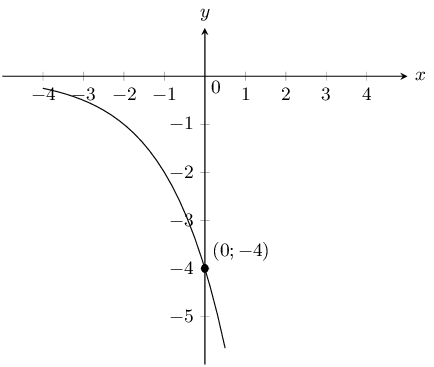$$y=\left(\frac{1}{3}\right)^{x} - 2$$

The $$y$$-intercept is $$(0;-2)$$. The $$x$$-intercept is $$(\text{0,6};0)$$. The asymptote is at $$y = -2$$.

$$0 < a < 1$$ therefore the graph curves downwards.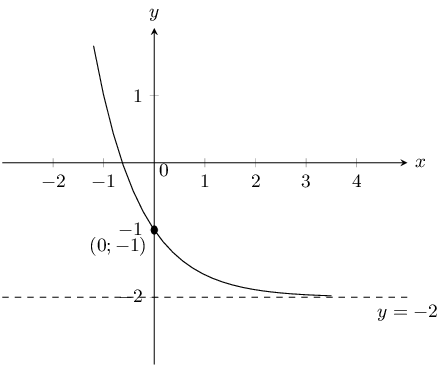The form of the curve graphed below is $$y = a \cdot \text{2}^{x} + q$$. One point is given on the curve: Point A is at $$(-\text{3};-\text{3,625})$$. Find the values of $$a$$ and $$q$$, correct to the nearest integer.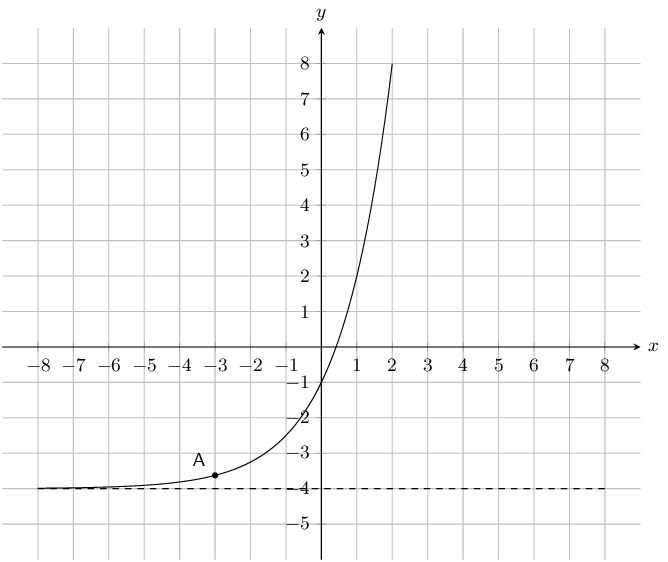The asymptote lies at $$y = -\text{4}$$. Therefore $$q$$ is $$-\text{4}$$.

At this point we know that the equation for the graph must be $$y = a.\text{2}^{x} -4$$.

\begin{align*} y & = a (\text{2})^{x} -\text{4} \\ (-\text{3,625}) & = a (\text{2})^{ (-\text{3})} -\text{4} \\ -\text{3,625} +4 & = a (\text{2})^{\left( -\text{3} \right)} \\ \text{0,375} & = a (\text{0,125}) \\ \text{3} & = a \end{align*} $$a = 3 \text{ and } q = -4$$

Given the following graph, identify a function that matches each of the given equations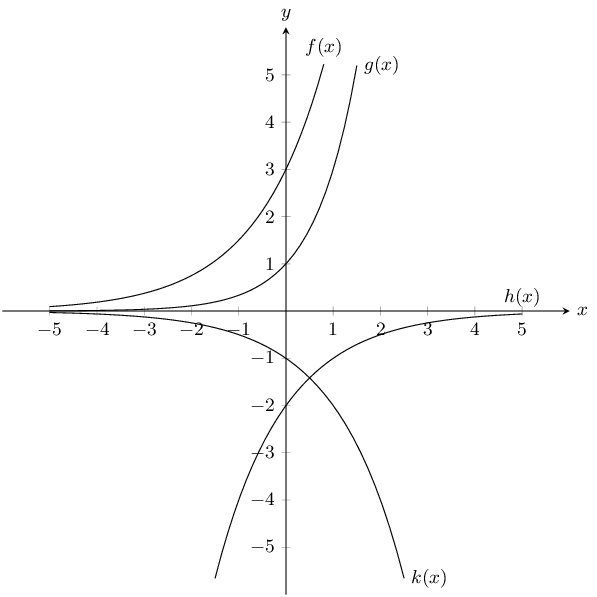$$y = -2\left(\dfrac{1}{2}\right)^{x}$$

$$h(x)$$

$$y = \text{3,2}^x$$

$$f(x)$$

$$y = -2^x$$

$$k(x)$$

$$y = 3^x$$

$$g(x)$$

Use the functions $$f(x) = 3 - x, g(x) = 2x^2 - 4; h(x) = 3^x - 4; k(x) = \dfrac{3}{2x} - 1,$$ to find the value of the following:

$$f(7)$$
\begin{align*} f(1) &= 3 - (7) \\ &= -4 \end{align*}
$$g(1)$$
\begin{align*} g(1) &= 2(1)^2 - 4 \\ &= -2 \end{align*}
$$h(-4)$$
\begin{align*} h(-4) &= 3^{-4} - 4 \\ &= -\frac{323}{81} \end{align*}
$$k(5)$$
\begin{align*} k(5) &= \frac{3}{2(5)} - 1\\ &= -\frac{7}{10} \end{align*}
$$f(-1) + h(-3)$$
\begin{align*} f(-1) + h(-3) &= 3 -(-1) + 3^{-3} - 4\\ &= \frac{1}{27} \end{align*}
$$h(g(-2))$$
\begin{align*} g(-2) &= 2(-2)^2 - 4 \\ &= 4 \\ \therefore h(g(-2)) &= h(4) \\ &= 3^{4} - 4 \\ &= 77 \end{align*}
$$k(f(6))$$
\begin{align*} f(6) &= 3 - (6) \\ &= -3 \\ \therefore k(f(6)) &= k(-3) \\ &= \frac{3}{2(-3)} -1 \\ &= -\frac{3}{2} \end{align*}

Determine whether the following statements are true or false. If the statement is false, give reasons why.

The given or chosen $$y$$-value is known as the independent variable.

False, the given or chosen $$y$$-value is the dependent variable because it's value depends on the independent variable $$x$$.

A graph is said to be continuous if there are breaks in the graph.

False, a graph is said to be continuous if there are no breaks in it.

Functions of the form $$y=ax+q$$ are straight lines.

True

Functions of the form $$y=\frac{a}{x}+q$$ are exponential functions.

False, functions of the form $$y=\frac{a}{x}+q$$ are hyperbolic functions.

An asymptote is a straight line which a graph will intersect at least once.

False, an asymptote is a straight line that a graph will never intersect.

Given a function of the form $$y=ax+q$$, to find the $$y$$-intercept let $$x=0$$ and solve for $$y$$.

True

Given the functions $$f(x) = 2x^{2}-6$$ and $$g(x)=-2x+6$$.

Draw $$f$$ and $$g$$ on the same set of axes.

For $$g(x)$$ the $$y$$-intercept is $$(0;6)$$ and the $$x$$-intercept is $$(3;0)$$.

For $$f(x)$$ the $$y$$-intercept is $$(0;-6)$$ and the $$x$$-intercepts are $$\left(\sqrt{3};0\right)$$ and $$\left(-\sqrt{3};0\right)$$.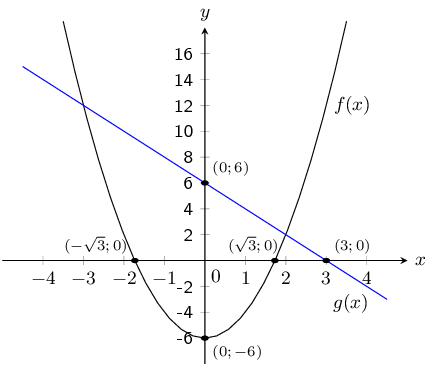Calculate the points of intersection of $$f$$ and $$g$$.

The $$x$$-values of the points of intersection can be found by setting $$f(x)=g(x)$$:

\begin{align*} 2x^2 - 6 &= -2x +6\\ 2x^2+2x-12&=0\\ x^2 +x - 6&=0\\ (x-2)(x+3) &= 0\\ \therefore x=2 &\text{ and }x=-3 \end{align*}

The $$y$$-values can be obtained by substituting the $$x$$-values into either equation:

\begin{align*} g(x) &= -2(-3)+6 = 12\\ g(x) &= -2(2)+6=2 \end{align*}

Therefore the points of intersection are $$(-3;12)$$ and $$(2;2)$$.

Use your graphs and the points of intersection to solve for $$x$$ when:

1. $$f(x) > 0$$
2. $$g(x) < 0$$
3. $$f(x) \le g(x)$$
1. \begin{align*} \text{Let }f(x)&=0\\ 2x^2-6&=0\\ 2x^2&=6\\ x^2&=3\\ x&=\pm \sqrt{3} \end{align*}

Therefore, for $$f(x)>0$$, $$x \in (-\infty; \sqrt{3})\cup(\sqrt{3}; \infty)$$.

2. \begin{align*} \text{Let }g(x)&=0\\ -2x+6&=0\\ -2x&=-6\\ x&=3 \end{align*}

Therefore, for $$g(x) <0$$, $$x \in (3;\infty)$$.

3. This is found by looking at where the graph of $$f(x)$$ lies underneath the graph of $$g(x)$$.

For $$f(x)\le g(x)$$, $$x \in [-3;2]$$.

Give the equation of the reflection of $$f$$ in the $$x$$-axis.

$$y=-2x^2+6$$

After a ball is dropped, the rebound height of each bounce decreases. The equation $$y=5(\text{0,8})^{x}$$ shows the relationship between the number of bounces $$x$$ and the height of the bounce $$y$$ for a certain ball. What is the approximate height of the fifth bounce of this ball to the nearest tenth of a unit?

For the fifth bounce $$x = 5$$. Now we can solve for $$y$$:

\begin{align*} y &= 5(\text{0,8})^x\\ & = 5\left(\frac{4}{5}\right)^5\\ &= 5\left(\frac{1024}{3125}\right)\\ &=5(\text{0,38})\\ &=\text{1,6} \text{ units} \end{align*}

Therefore the approximate height of the fifth bounce is $$\text{1,6}$$ units

Mark had $$\text{15}$$ coins in $$\text{R}\,\text{5}$$ and $$\text{R}\,\text{2}$$ pieces. He had $$\text{3}$$ more $$\text{R}\,\text{2}$$ coins than $$\text{R}\,\text{5}$$ coins. He wrote a system of equations to represent this situation, letting $$x$$ represent the number of $$\text{R}\,\text{5}$$ coins and $$y$$ represent the number of $$\text{R}\,\text{2}$$ coins. Then he solved the system by graphing.

Write down the system of equations.

Let $$x = \text{R}\,\text{5}$$ coins and $$y = \text{R}\,\text{2}$$ coins. Then the system of equations is:

$$x+y=15$$; $$y=x+3$$

Draw their graphs on the same set of axes.

For $$x + y = 15$$ the $$y$$-intercept is $$(0;15)$$ and the $$x$$-intercept is $$(15;0)$$.

For $$y = x + 3$$ the $$y$$-intercept is $$(0;3)$$ and the $$x$$-intercept is $$(-3;0)$$.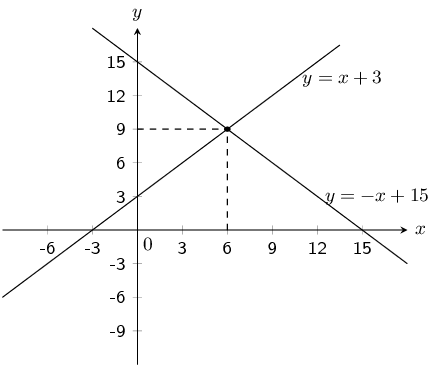Use your sketch to determine how many $$\text{R}\,\text{5}$$ and $$\text{R}\,\text{2}$$ pieces Mark had.

From the sketch we see that the graphs intersect at $$(6;9)$$. Checking algebraically we get:

Substitute the value of $$y=-x+15$$ into the second equation:

\begin{align*} -x+15&=x+3\\ -2x &= -12\\ \therefore x&=6 \end{align*}

Substitute the value of $$x$$ back into the first equation:

\begin{align*} y &= -(6) + 15\\ &= 9 \end{align*}

Mark has $$\text{6}$$ $$\text{R}\,\text{5}$$ coins and $$\text{9}$$ $$\text{R}\,\text{2}$$ coins.

Shown the following graph of the following form: $$y = a \sin{\theta} + q$$ where Point A is at $$(90^{\circ};\text{4,5})$$, and Point B is at $$(180^{\circ};3)$$, determine the values of $$a$$ and $$q$$.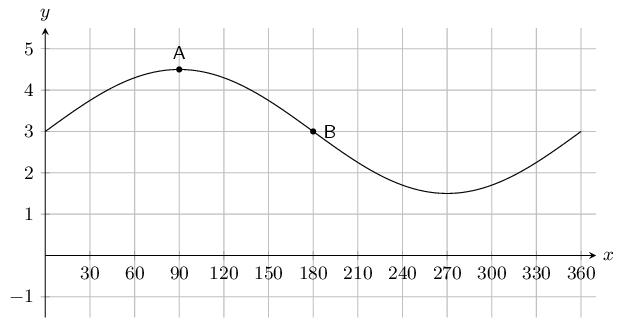To find $$q$$ we note that $$q$$ shifts the graph up or down. To determine $$q$$ we can look at any point on the graph. For instance point $$B$$ is at $$(180^{\circ};3)$$. For an unshifted sine graph point $$B$$ would be at $$(180^{\circ};0)$$. For this graph we see that this point has been shifted up by 3 spaces. Therefore $$q = 3$$.

To find $$a$$ we note that the $$y$$-value at the middle (point $$B$$) is 3, while the $$y$$-value at the top (point $$A$$) is $$\text{4,5}$$. We can find the amplitude by working out the distance from the top of the graph to the middle of the graph: $$\text{4,5} - 3 = \text{1,5}$$. Therefore $$a = \frac{3}{2}$$.

The complete equation for the graph shown in this question is $$y = \frac{3}{2}\sin \theta + 3$$.

Therefore $$a = \frac{3}{2} \text{ and } q = 3$$.

The graph below shows a trigonometric equation of the following form: $$y = a \cos{\theta} + q$$. Two points are shown on the graph: Point A at $$(90^{\circ};0)$$, and Point B: $$(180^{\circ};-\text{3})$$. Calculate the values of $$a$$ (the amplitude of the graph) and $$q$$ (the vertical shift of the graph).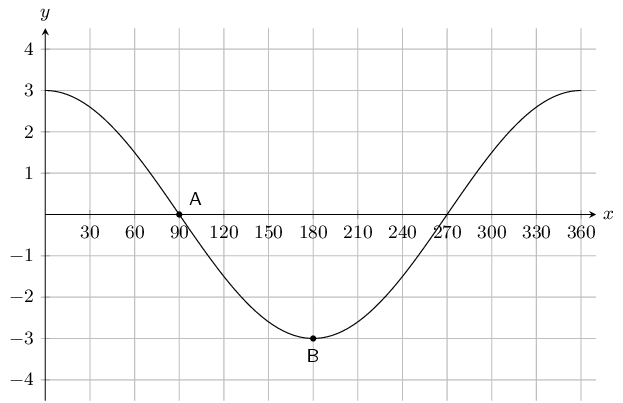To find $$q$$ we note that $$q$$ shifts the graph up or down. To determine $$q$$ we can look at any point on the graph. For instance point $$A$$ is at $$(90^{\circ};0)$$. For an unshifted cosine graph point $$A$$ would be at $$(90^{\circ};0)$$. For this graph we see that this point has not been shifted. Therefore $$q = 0$$.

To find $$a$$ we note that the $$y$$-value at the middle (point $$A$$) is 0, while the $$y$$-value at the bottom (point $$B$$) is $$-\text{3}$$. We can find the amplitude by working out the distance from the middle of the graph to the bottom of the graph: $$0 - (-3) = 3$$. Therefore $$a = 3$$.

The complete equation for the graph shown in this question is $$y = 3\cos \theta$$.

Therefore $$a = 3 \text{ and } q = 0$$.

On the graph below you see a tangent curve of the following form: $$y = a \tan{\theta} + q$$. Two points are labelled on the curve: Point A is at $$\left(0^{\circ} ; -3 \right)$$, and Point B is at $$\left(45^{\circ} ; -2 \right)$$.

Calculate, or otherwise determine, the values of $$a$$ and $$q$$.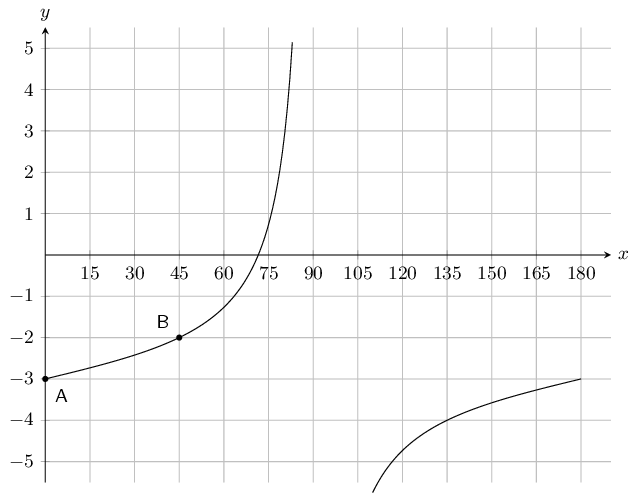To find $$q$$ we note that $$q$$ shifts the graph up or down. To determine $$q$$ we can look at any point on the graph. For instance point $$A$$ is at $$\left(0^{\circ};-3\right)$$. For an unshifted tangent graph point $$A$$ would be at $$(0^{\circ};0)$$. For this graph we see that this point been shifted downwards by $$3$$. Therefore $$q = -3$$.

To find $$a$$ we can substitute point $$B$$ into the equation for the tangent graph:

\begin{align*} y & = a \tan{\theta} -3 \\ \left( -2 \right) & = a \tan{45^{\circ}} - 3 \\ -2 & = a(-1) - 3 \\ -2 + 3 & = -a \\ -1 & = -a \\ 1 & = a \end{align*}

The complete equation is: $$y = \tan{\theta} - 3$$.

Therefore $$a = 1 \text{ and } q = -3$$.

Given the following graph, identify a function that matches each of the given equations: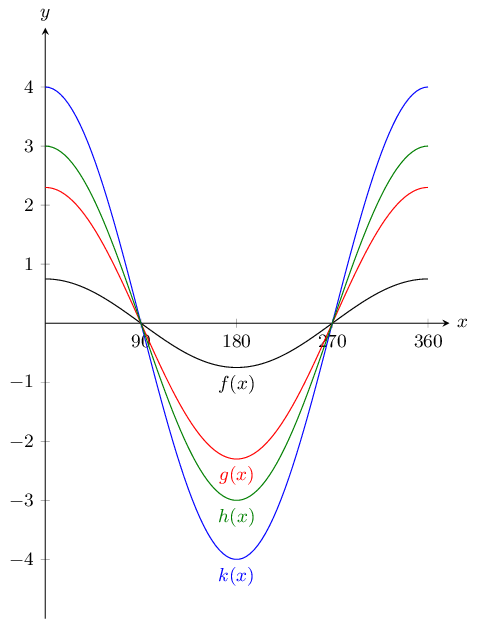$$y = \text{2,3}\cos{\theta}$$

$$g(x)$$

$$y = \text{0,75}\cos{\theta}$$

$$f(x)$$

$$y = 4\cos{\theta}$$

$$k(x)$$

$$y = 3\cos{\theta}$$

$$h(x)$$

The graph below shows functions $$f(x)$$ and $$g(x)$$.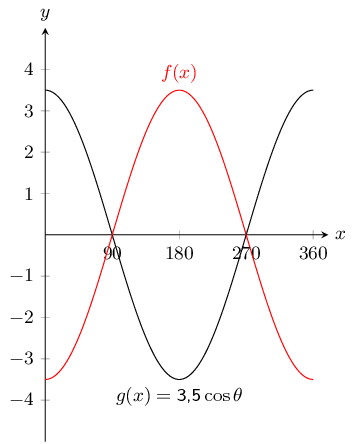What is the equation for $$f(x)$$?

$$f(x) = -\text{3,5}\cos{\theta}$$

With the assistance of the table below sketch the three functions on the same set of axes.

$\begin{array}{| l | l | l | l | l | l | l | l | l | l | } \hline \theta & 0^{\circ} & 45^{\circ} & 90^{\circ} & 135^{\circ} & 180^{\circ} & 225^{\circ} & 270^{\circ} & 315^{\circ} & 360^{\circ} \\ \hline \tan{\theta} & 0 & 1 & \text{undefined} & -1 & 0 & 1 & \text{undefined} & -1 & 0 \\ \hline 2\tan{\theta} & 0 & 2 & \text{undefined} & -2 & 0 & 2 & \text{undefined} & -2 & 0 \\ \hline \frac{1}{3}\tan{\theta} & 0 & \frac{1}{3} & \text{undefined} & -\frac{1}{3} & 0 & \frac{1}{3} & \text{undefined} & -\frac{1}{3} & 0 \\ \hline \end{array}$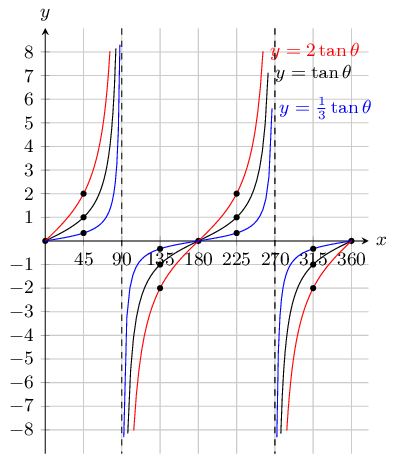With the assistance of the table below sketch the three functions on the same set of axes.

$\begin{array}{| l | l | l | l | l | l | } \hline \theta & 0^{\circ} & 90^{\circ} & 180^{\circ} & 270^{\circ} & 360^{\circ} \\ \hline \sin{\theta} + 1 & 1 & 2 & 1 & 0 & 1 \\ \hline \sin{\theta} + 2 & 3 & 2 & 2 & 1 & 2 \\ \hline \sin{\theta} - 2 & -2 & -1 & -2 &-3 & -2 \\ \hline \end{array}$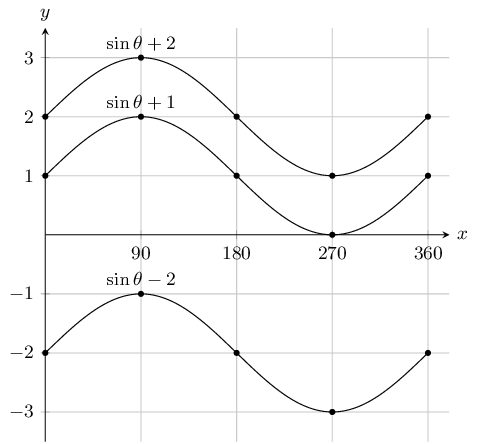Sketch graphs of the following trigonometric functions for $$\theta \in \left[0°;360°\right]$$. Show intercepts and asymptotes.

$$y=-4\cos \theta$$

The $$y$$-intercept is $$(0^\circ;-4)$$. The $$x$$-intercepts are $$(90^\circ;0)$$ and $$(270^\circ;0)$$. There are no asymptotes.

The graph is not shifted up or down since $$q = 0$$. The graph is stretched by 4 and reflected in the $$x$$-axis.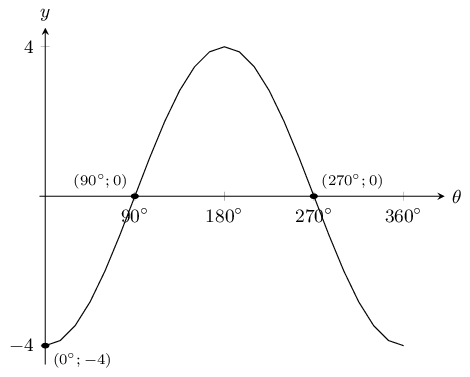$$y=\sin \theta - 2$$

The $$y$$-intercept is $$(0^\circ;-2)$$. There are no $$x$$-intercepts. There are no asymptotes.

The graph is shifted down by $$-\text{2}$$ since $$q = -2$$. The graph is not stretched since $$a = 1$$.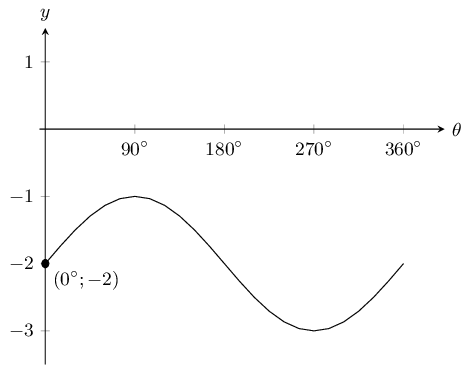$$y=-2\sin \theta + 1$$

The $$y$$-intercept is $$(0^\circ;1)$$. The $$x$$-intercepts are $$(30^\circ;0)$$ and $$(150^\circ;0)$$. There are no asymptotes.

The graph is shifted up by 1 since $$q = 1$$. The graph is stretched by $$-\text{2}$$ and reflected in the $$x$$-axis since $$a = -2$$.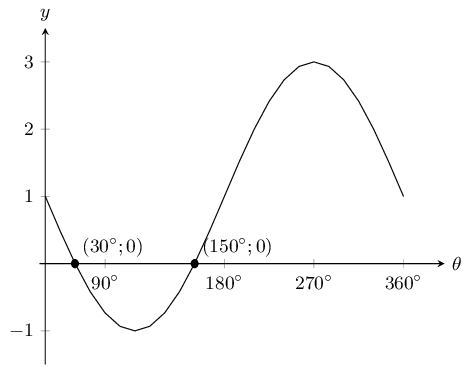$$y=\tan \theta + 2$$

The $$y$$-intercept is $$(0^\circ;2)$$. The $$x$$-intercepts are $$(\text{116,57}^\circ;0)$$ and $$(\text{296,57}^\circ;0)$$. The asymptotes are $$x = 90^\circ$$ and $$x = 270^\circ$$.

The graph is shifted up by 2 since $$q = 2$$. The graph is not stretched since $$a = 1$$.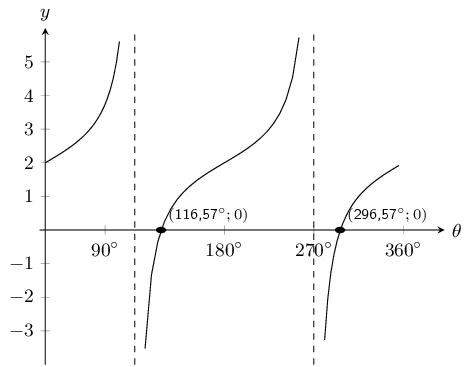$$y=\frac{\cos \theta}{2}$$

The $$y$$-intercept is $$(0^\circ;\text{0,5})$$. The $$x$$-intercepts are $$(90^\circ;0)$$ and $$(270^\circ;0)$$. There are no asymptotes.

The graph is not shifted upwards or downwards since $$q = 0$$. The graph is stretched by $$\text{0,5}$$ since $$a = \frac{1}{2}$$.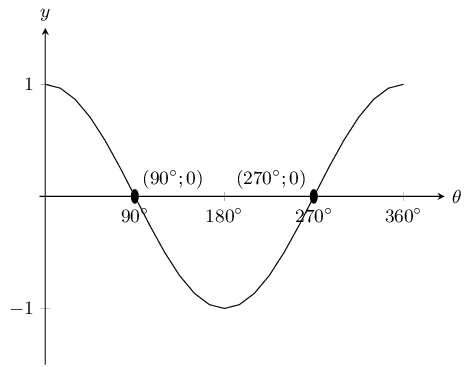State the coordinates at $$E$$ and the range of the function.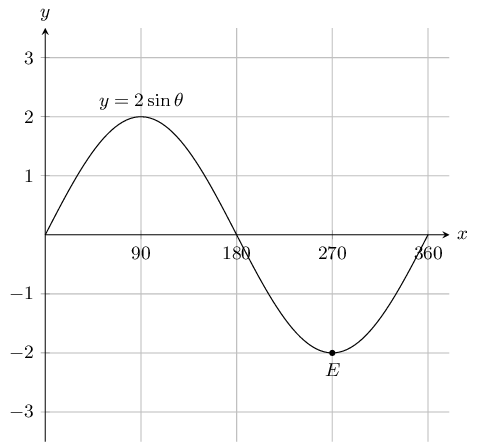$$E = (270^{\circ};-2) \text{ and } -2 \le y \le 2$$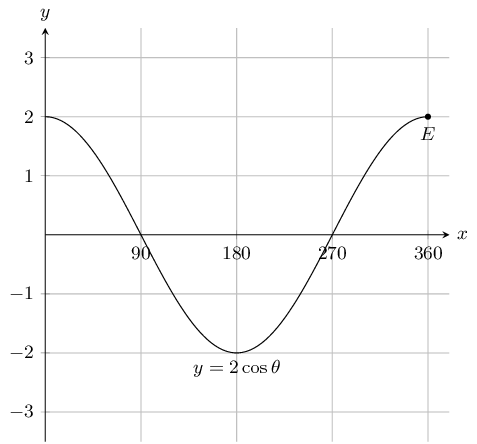$$E = (360^{\circ};2) \text{ and } -2 \le y \le 2$$

State the coordinates at $$E$$ and the domain and range of the function in the interval shown.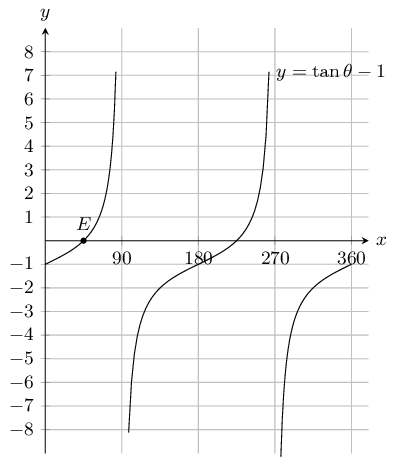$$E = (45^{\circ};0) \text{ , range } y \in \mathbb{R} \text{ and domain } 0 \le x \le 360, x \neq 90, \theta \neq 270$$

For which values of $$\theta$$ is the function decreasing, in the interval shown?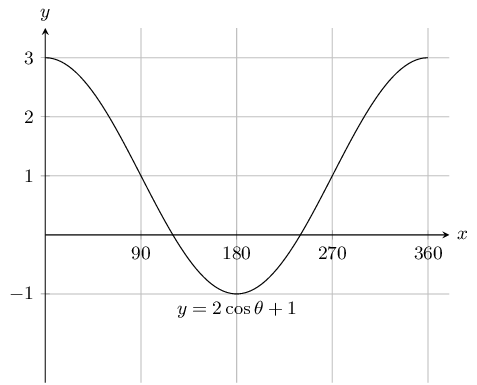$$0^{\circ} < \theta < 180^{\circ}$$

For which values of $$\theta$$ is the function increasing, in the interval shown?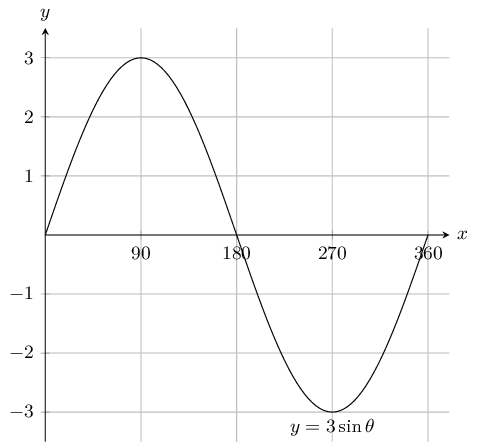$$0^{\circ} < \theta < 90^{\circ} \text{ and } 270^{\circ} < \theta < 360^{\circ}$$

For which values of $$\theta$$ is the function positive, in the interval shown?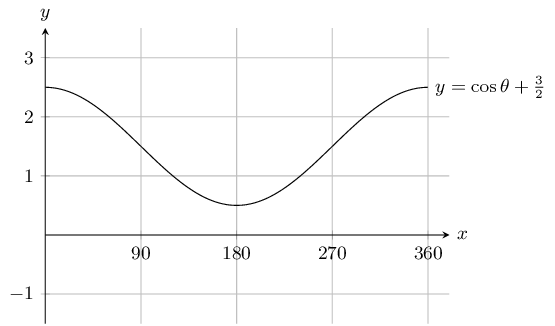$$0^{\circ} < \theta < 360^{\circ}$$

Given the general equations $$y=mx+c$$, $$y=ax^2+q$$, $$y=\dfrac{a}{x} + q$$, $$y = a \cdot b^x + q$$, $$y=a\sin \theta+q$$, $$y=a\cos \theta+q$$ and $$y=a\tan \theta$$, determine the specific equations for each of the following graphs.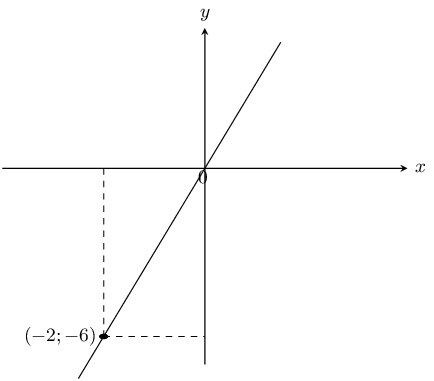This is a straight line graph and so the general equation is $$y = mx + c$$. The $$y$$-intercept is at $$(0;0)$$ and so $$c = 0$$.

To find $$m$$ we substitute in the given point into the equation and solve for $$m$$:

\begin{align*} y & = mx \\ -6 & = -2m \\ m & = 3 \end{align*}

Therefore the equation is $$y=3x$$.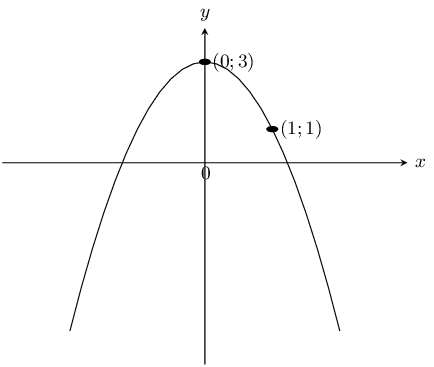This is a parabola and so we use $$y = ax^2 + q$$. The $$y$$-intercept is at $$(0;3)$$ and so $$q = 3$$.

We substitute the point $$(1;1)$$ into the equation and solve for $$a$$:

\begin{align*} y & = ax^2 + 3 \\ 1 & = a(1)^2 + 3 \\ -2 & = a \end{align*}

Therefore the equation is $$y=-2x^{2}+3$$.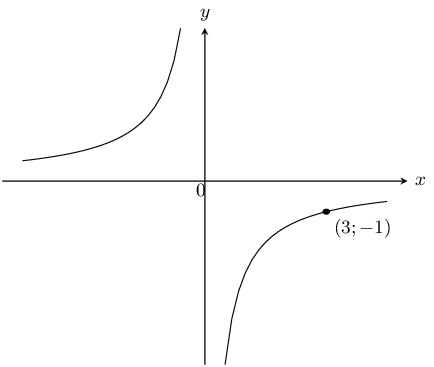This is a hyperbola and so we use $$y = \frac{a}{x} + q$$. There is no $$x$$-intercept and so the graph has not been shifted upwards or downwards. Therefore $$q = 0$$.

We substitute the point $$(3;-1)$$ into the equation and solve for $$a$$:

\begin{align*} y & = \frac{a}{x} \\ -1 & = \frac{a}{3} \\ -3 & = a \end{align*}

Therefore the equation is $$y=\frac{-3}{x}$$.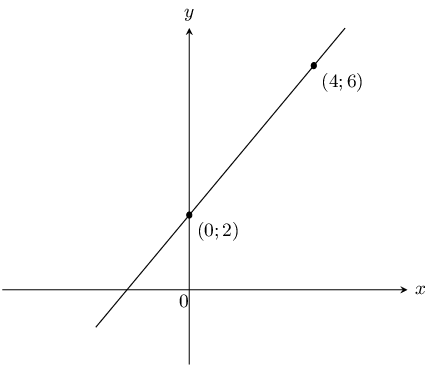This is a straight line graph and so the general equation is $$y = mx + c$$. The $$y$$-intercept is at $$(0;2)$$ and so $$c = 2$$.

To find $$m$$ we substitute the point $$(4;6)$$ into the equation and solve for $$m$$:

\begin{align*} y & = mx + 2 \\ 6 & = 4m + 2 \\ m & = 1 \end{align*}

Therefore the equation is $$y=x + 2$$.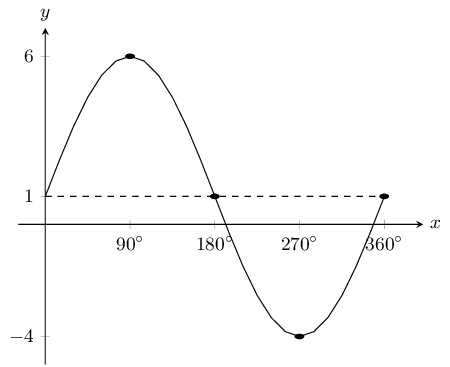This is a sine graph and so the general equation is $$y = a\sin\theta + q$$.

To find $$a$$ we note that the $$y$$-value at the bottom is $$-\text{4}$$, while the $$y$$-value at the top is $$\text{6}$$. We can find the amplitude by working out the distance from the bottom of the graph to the top of the graph and dividing this value by 2: $$\frac{6 - (-4)}{2} = 5$$. Therefore $$a = 5$$.

To find $$q$$ we note that $$q$$ shifts the graph up or down. To determine $$q$$ we can look at any point on the graph. For instance we can see that when $$x = 180^\circ$$, $$y = 1$$. For an unshifted sine graph with the same $$a$$ value (i.e. $$5 \sin \theta$$) this point would be at $$(180^{\circ};0)$$. For this graph we see that this point has been shifted upwards by 1 unit. Therefore $$q = 1$$.

The complete equation for the graph shown in this question is $$y = 5\sin \theta + 1$$.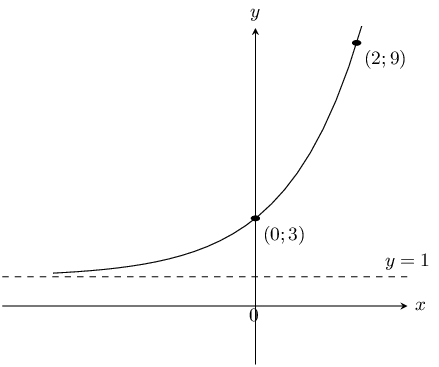This is an exponential graph and so we use $$y = a \cdot b^x + q$$. We see that the asymptote is at $$y = 1$$ and so $$q = 1$$. To find $$a$$ we substitute the point $$(0;3)$$ into the equation:

\begin{align*} y & = a \cdot b^x + 1 \\ 3 & = a \cdot b^0 + 1 \\ a & = 2 \end{align*}

To find $$b$$ we substitute the point $$(2;9)$$ into the equation:

\begin{align*} y & = 2 \cdot b^x + 1 \\ 9 & = 2 \cdot b^2 + 1 \\ 4 & = b^2 \\ 2^2 & = b^2 \\ b & = 2 \end{align*}

Therefore the equation is $$y=2 \times 2^{x} + 1$$.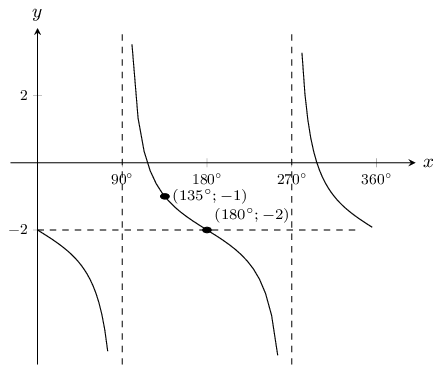This is a tangent graph and so we use $$y = a \tan \theta + q$$. To find $$q$$ we note that the graph has been shifted down by 2 units (the point $$(180^\circ;-2)$$ is given). Therefore $$q = -2$$.

To find $$a$$ we substitute $$(135^\circ;-1)$$ into the equation:

\begin{align*} y & = a \tan \theta - 2 \\ -1 & = a \tan (135^\circ) - 2 \\ 1 & = -a \\ a & = -1 \end{align*}

Therefore the equation is $$y=-\tan \theta - 2$$.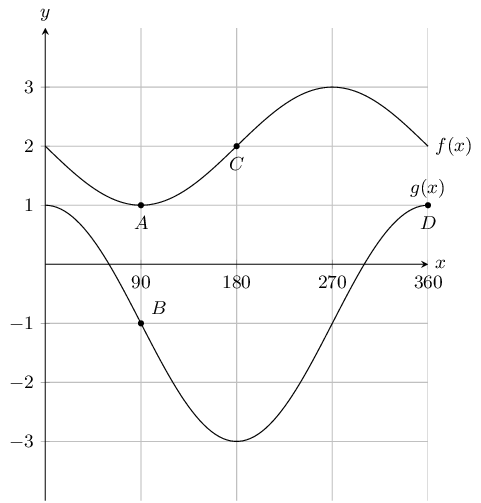State the coordinates at $$A,~B,~C$$ and $$D$$.

$$A( 90^{\circ};1 ),~B(90 ^{\circ};-1 ),~C( 180^{\circ}; 2) \text{ and } D(360 ^{\circ};1)$$

How many times in this interval does $$f(x)$$ intersect $$g(x)$$.

0

What is the amplitude of $$f(x)$$.

1

Evaluate: $$f(180^{\circ}) - g(180^{\circ})$$ .

\begin{align*} f(180^{\circ}) - g(180^{\circ}) &= 2 - (-3)\\ &=5 \end{align*}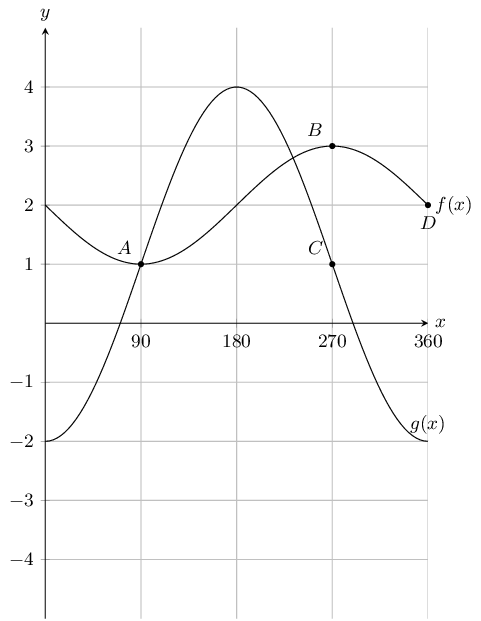State the coordinates at $$A,~B,~C$$ and $$D$$.

$$A( 90^{\circ}; 1),~B(270 ^{\circ}; 3),~C(270 ^{\circ}; 1) \text{ and } D(360 ^{\circ}; 2)$$

How many times in this interval does $$f(x)$$ intersect $$g(x)$$.

2

What is the amplitude of $$g(x)$$.

3

Evaluate: $$g(180^{\circ}) - f(180^{\circ})$$ .

\begin{align*} f(180^{\circ}) - g(180^{\circ}) &= 4 - 2 \\ &=2 \end{align*}

$$y=2^{x}$$ and $$y=-2^{x}$$ are sketched below. Answer the questions that follow.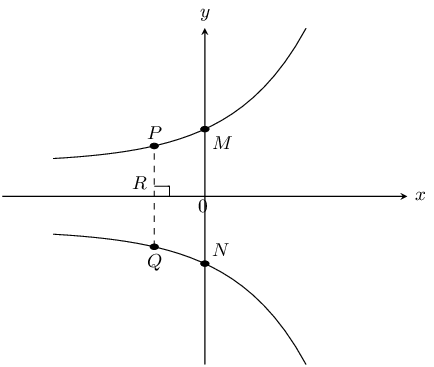Calculate the coordinates of $$M$$ and $$N$$.

$$M$$ is the $$y$$-intercept of $$y=2^{x}$$ and so $$y = 2^0 = 1$$. Therefore the coordinates of $$M$$ are $$(0;1)$$.

$$N$$ is the $$y$$-intercept of $$y=-2^{x}$$ and so $$y= -(2^0)= -1$$. Therefore the coordinates of $$N$$ are $$(0;-1)$$.

Therefore $$M(0;1)$$ and $$N(0;-1)$$.

Calculate the length of $$MN$$.

$$M$$ and $$N$$ both lie on the $$y$$-axis and so they both lie on a straight line.

Therefore $$MN = 1 + 1 = 2$$.

Calculate the length of $$PQ$$ if $$OR = \text{1}\text{ unit}$$.

At $$P$$, $$x=-1$$, therefore $$y = 2^{-1} = \frac{1}{2}$$.

At $$Q$$, $$x=-1$$, therefore $$y=-(2^{-1}) = -\frac{1}{2}$$.

Therefore length $$PQ = \frac{1}{2} + \frac{1}{2} =1$$.

Give the equation of $$y=2^{x}$$ reflected about the $$y$$-axis.

$$y=2^{-x}$$

Give the range of both graphs.

Range $$y=2^x$$: $$(0;\infty)$$

Range $$y=-2^x$$: $$(-\infty;0)$$

Plot the following functions on the same set of axes and clearly label all points of intersection.

\begin{aligned} y & = -2x^2 +3 \\ y & = 2x + 4 \end{aligned}

For $$y = -2x^2 + 3$$:

The $$y$$-intercept is at $$(0;3)$$. The $$x$$-intercepts are at $$\left(\sqrt{\frac{3}{2}};0\right)$$ and $$\left(-\sqrt{\frac{3}{2}};0\right)$$.

For $$y = 2x + 4$$:

The $$y$$-intercept is at $$(0;4)$$. The $$x$$-intercept is at $$(-2;0)$$.

There are no points of intersection.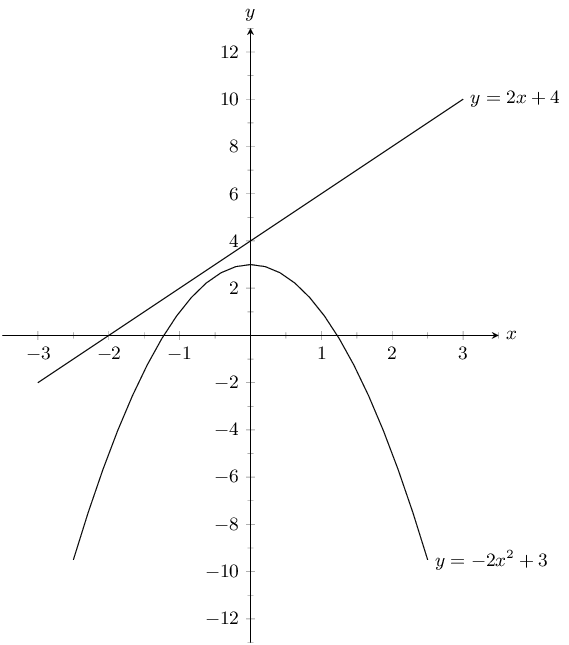\begin{aligned} y & =x^2 -4 \\ y & = 3x \end{aligned}

For $$y = x^2 - 4$$:

The $$y$$-intercept is at $$(0;-4)$$. The $$x$$-intercepts are at $$(2;0)$$ and $$(-2;0)$$.

For $$y = 3x$$:

The $$y$$-intercept is at $$(0;0)$$. The $$x$$-intercept is at $$(0;0)$$.

To find the point of intersection we equate the two functions:

\begin{align*} x^2 - 4 &= 3x \\ x^2 - 3x - 4 &= 0 \\ (x - 4)(x + 1) & = 0\\ x = 4 &\text{ or } x = -1 \\ y = 3(4) \text{ or } y = 3(-1) \\ y = 12 \text{ or } y = -3 \end{align*}

Therefore the graphs intersect at $$(4;12)$$ and $$(-1;-3)$$.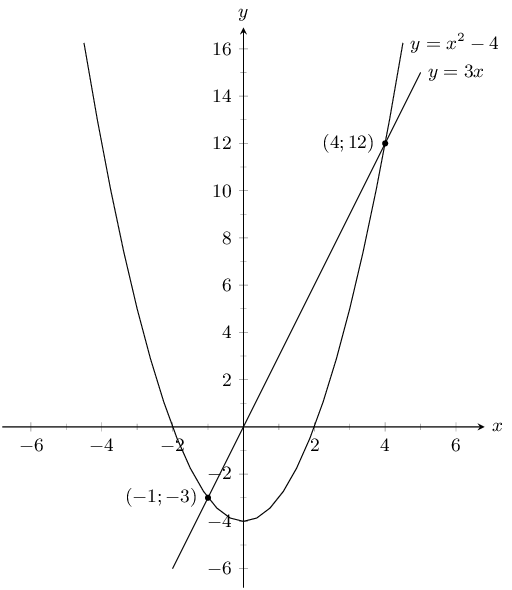$$f(x)=4^x$$ and $$g(x)=-4x^2+q$$ are sketched below. The points $$A(0;1)$$ and $$B(1;4)$$ are given. Answer the questions that follow.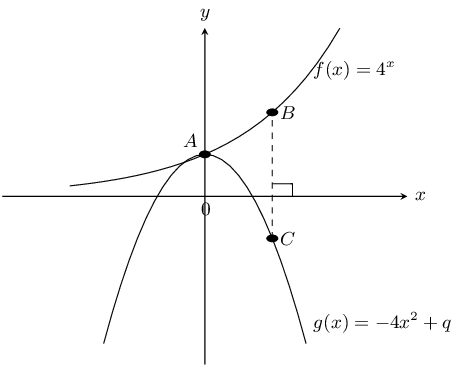Determine the value of $$q$$.

Point $$A$$ is the $$y$$-intercept of $$g(x)$$ and so $$q=1$$.

Calculate the length of $$BC$$.

$$B$$ is at $$(1;4)$$ and so $$C$$ is at $$(1;y)$$. To find $$y$$ we substitute point $$C$$ into $$g(x)$$:

\begin{align*} g(x) & = -4x^2 + 1 \\ y & = -4(1)^2 + 1 \\ & = -3 \end{align*}

Therefore $$BC = 3 + 4 = \text{7}\text{ units}$$.

Give the equation of $$f(x)$$ reflected about the $$x$$-axis.

$$y=-4^{x}$$

Give the equation of $$f(x)$$ shifted vertically upwards by $$\text{1}$$ unit.

$$y=4^{x}+1$$

Give the equation of the asymptote of $$f(x)$$.

$$y=0$$

Give the ranges of $$f(x)$$ and $$g(x)$$.

Range $$f(x)$$: $$(0;\infty)$$, Range $$g(x)$$: $$(-\infty;1]$$

Given $$h(x)=x^2-4$$ and $$k(x)=-x^2+4$$. Answer the questions that follow.

Sketch both graphs on the same set of axes.

For $$h(x)$$ the $$y$$-intercept is at $$(0;4)$$. The $$x$$-intercepts are at $$(2;0)$$ and $$(-2;0)$$.

For $$k(x)$$ the $$y$$-intercept is at $$(0;-4)$$. The $$x$$-intercepts are at $$(2;0)$$ and $$(-2;0)$$.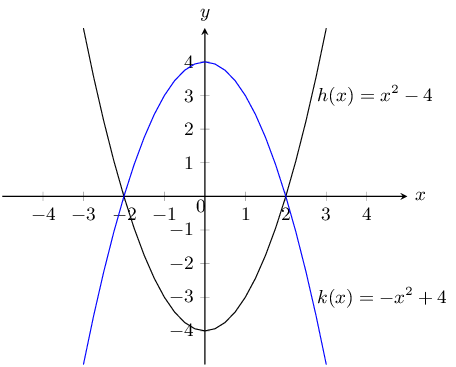Describe the relationship between $$h$$ and $$k$$.

\begin{align*} h(x) &= x^2 - 4\\ k(x) &= -x^2+4\\ &=-(x^2-4)\\ &= -h(x) \end{align*}

$$k(x)$$ is therefore the reflection of $$h(x)$$ about the $$x$$-axis.

Give the equation of $$k(x)$$ reflected about the line $$y=4$$.

$$y=x^2+4$$

Give the domain and range of $$h$$.

Domain $$h$$: $$(-\infty;\infty)$$. Range $$h$$: $$[-4;\infty)$$.

Sketch the graphs of $$f(\theta)=2\sin \theta$$ and $$g(\theta)=\cos \theta-1$$ on the same set of axes. Use your sketch to determine:

1. $$f(180°)$$
2. $$g(180°)$$
3. $$g(270°) - f(270°)$$
4. The domain and range of $$g$$.
5. The amplitude and period of $$f$$.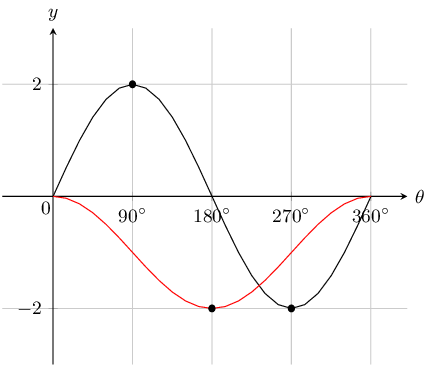1. $$f(180°)=0$$

2. $$g(180°)=-2$$

3. $$g(270°)-f(270°)=-1-(-2)=-1+2=1$$

4. Domain: $$[0°;360°]$$. Range: $$[-2;0]$$

5. Amplitude: $$\text{2}$$. Period: $$360°$$

The graphs of $$y=x$$ and $$y=\dfrac{8}{x}$$ are shown in the following diagram.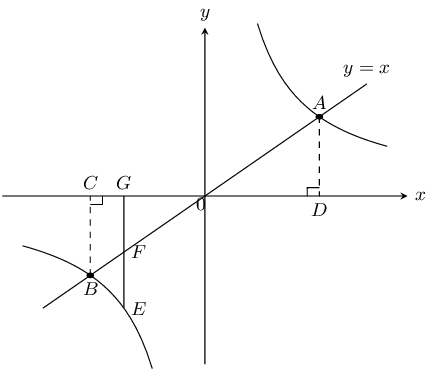Calculate:

The coordinates of points $$A$$ and $$B$$.

$$A$$ and $$B$$ are the points of intersection of the two functions. Therefore:

\begin{align*} x&=\frac{8}{x}\\ x^2&=8\\ \therefore x&=\pm\sqrt{8} \end{align*}

Since the equation of the straight line is $$y = x$$ these are also the $$y$$-values of the points of intersection.

Therefore $$A(\sqrt{8};\sqrt{8})$$ and $$B(-\sqrt{8};-\sqrt{8})$$

The length of $$CD$$.

$$C$$ has the same $$x$$ value as $$A$$ and $$D$$ has the same $$x$$ value as $$B$$.

Therefore $$C(-\sqrt{8};0)$$ and $$D(\sqrt{8};0)$$.

$$CD = \sqrt{8} +\sqrt{8} =2\sqrt{8}$$.

The length of $$AB$$.

Using Pythagoras:

\begin{align*} OD=\sqrt{8} \text{ units and }&AD=\sqrt{8} \text{ units}\\ AO^2&=OD^2+AD^2\\ &=(\sqrt{8})^2+(\sqrt{8})^2\\ &=8+8\\ &=16\\ \therefore AO&=4 \text{ units} \\ \text{Similarly, } OB&=4 \text{ units}\\ \therefore AB&=8 \text{ units} \end{align*}

The length of $$EF$$, given $$G(-2;0)$$.

$$F$$ and $$E$$ have the same $$x$$ value as point $$G$$. $$F$$ lies on $$y = x$$ and so $$F(-2;-2)$$. $$E$$ lies on $$y = \frac{8}{x}$$ and so $$E(-2;-4)$$.

Therefore length $$EF = 2 + 4 = 2$$ units.

Given the diagram with $$y=-3x^2+3$$ and $$y=-\dfrac{18}{x}$$.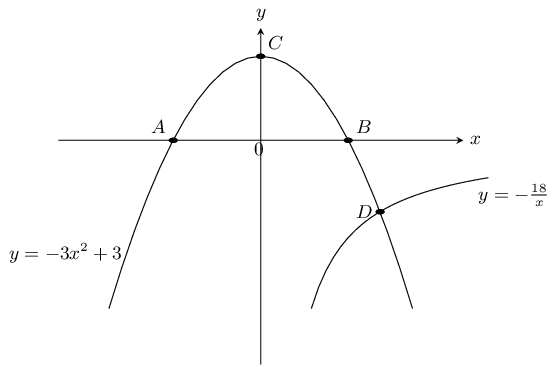Calculate the coordinates of $$A$$, $$B$$ and $$C$$.

$$A$$ and $$B$$ are the $$x$$-intercepts of $$y=-3x^2+3$$. $$C$$ is the $$y$$-intercept of $$y=-3x^2+3$$.

Therefore point $$C$$ is at $$(0;3)$$.

Points $$B$$ and $$A$$ are at $$(1;0)$$ and $$(-1;0)$$ respectively.

Therefore $$A(-1;0)$$, $$B(1;0)$$, $$C(0;3)$$

Describe in words what happens at point $$D$$.

The parabola and the hyperbola intersect at point $$D$$ which lies in the fourth quadrant.

Calculate the coordinates of $$D$$.

\begin{align*} -\frac{18}{x}&=-3x^2+3\\ -18&=-3x^3+3x\\ 0&=-3x^3 + 3x + 18\\ 0&=x^3 - x - 6\\ 0 & = (x - 2)(x^2 + 2x + 3) \\ x & = 2 \\ f(2)&=(2)^3-2-6=0\\ \text{when } x=2 \text{, } &y=-3(2)^2+3=-9\\ \therefore D(2;-9) \end{align*}

Determine the equation of the straight line that would pass through points $$C$$ and $$D$$.

Determine gradient $$D(2;-9)$$ and $$C(0;3)$$:

\begin{align*} m&=\frac{-9-3}{2-0} \\ & =-6\\ \end{align*}

$$C$$ is the $$y$$-intercept and so $$c = 3$$.

Therefore $$y=-6x+3$$.

The diagram shows the graphs of $$f(\theta)=3\sin\theta$$ and $$g(\theta)=-\tan\theta$$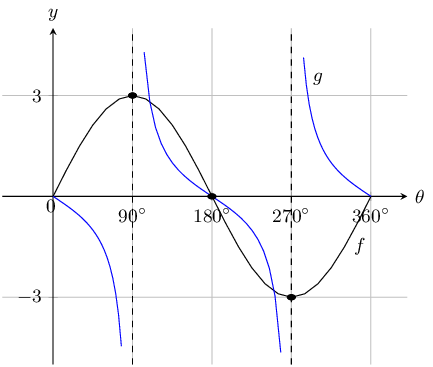Give the domain of $$g$$.

Domain: $$\{ \theta: 0° \le \theta \le 360°, \theta \ne 90°; 270° \}$$

What is the amplitude of $$f$$?

Amplitude: $$\text{3}$$

Determine for which values of $$\theta$$:

1. $$f(\theta)=0=g(\theta)$$
2. $$f(\theta) \times g(\theta) < 0$$
3. $$\frac{g(\theta)}{f(\theta)} > 0$$
4. $$f(\theta)$$ is increasing
1. $$\{0°;~180°;~360°\}$$

2. $$(0°;~90°) \cup (270°;~360°)$$

3. $$\{\theta: 90° < \theta <270°, \theta \ne 180°\}$$

4. $$(0°;~90°) \cup (270°;~360°)$$

Determine the equations for the graphs given below.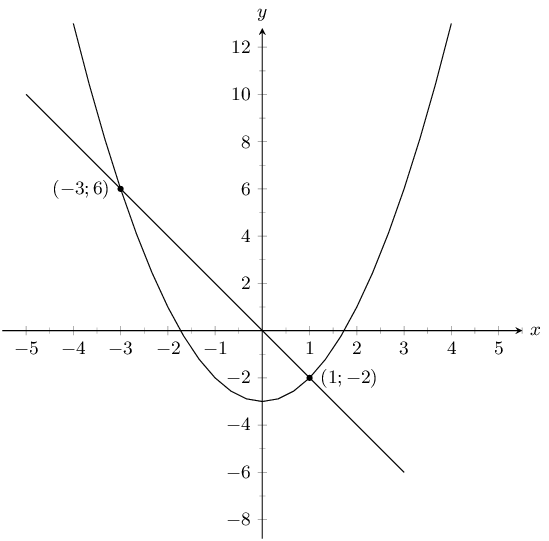For the straight line:

\begin{align*} y &= mx + c \\ c &= 0 \\ m &= \frac{6 - (-2)}{-3 - 1} \\ m &= -2 \\ y &= -2x \end{align*}

For the parabola:

\begin{align*} y &= ax^2 + q \\ q &= -3 \\ y &= ax^2 - 3 \\ 6 &= a(-3)^2 - 3 \\ 9 &= 9a \\ a &= 1 \\ y &= x^2 -3 \end{align*}

Therefore the equations are: $$y = -2x$$ and $$y = x^2 - 3$$.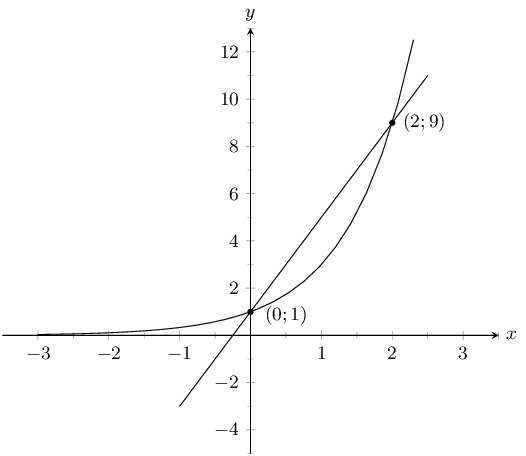For the straight line:

\begin{align*} y &= mx + c \\ c &= 1 \\ m &= \frac{9 - 1}{2 - 0} \\ m &= 4 \\ y &= 4x + 1 \end{align*}

For the exponential graph:

\begin{align*} y &= a \cdot b^x + q \\ q &= 0 \\ y &= a \cdot b^x \\ 1 &= a(b^0) \\ 1 &= a \\ y &= b^x \\ 9 &= b^2\\ 3^2 &= b^2\\ b &= 3\\ y &= 3^x \end{align*}

Therefore the equations are $$y = 4x + 1$$ and $$y = 3^x$$.

Which of the following does not have a gradient of 3?

1. $$y = 3x + 6$$
2. $$3y = 9x - 1$$
3. $$\frac{1}{3}(y - 1) = x$$
4. $$\frac{1}{2}(y - 3) = 6x$$


4

The asymptote of $$xy = 3 + x$$ is:

1. $$3$$
2. $$1$$
3. $$-3$$
4. $$-1$$


2

Sketch the following

$$y = -\text{1,5}^{x}$$

The asymptote is at $$y = 0$$. The $$y$$-intercept is at $$(0;-1)$$. There is no $$x$$-intercept.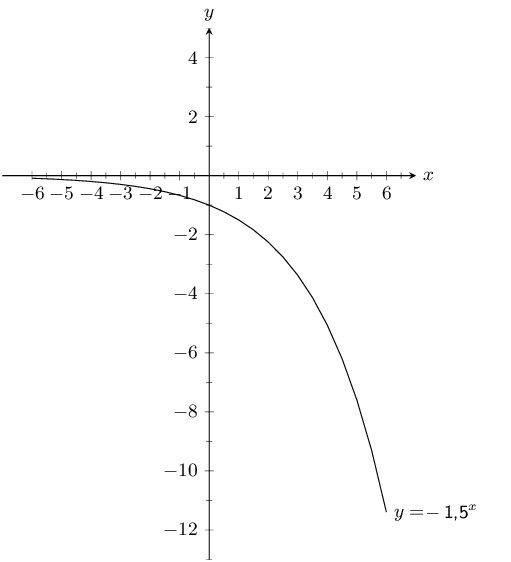$$xy = 5 + 2x$$

First rewrite the equation in standard form:

\begin{align*} xy & = 5 + 2x \\ y &= \frac{5}{x} + 2 \end{align*}

There is no $$y$$-intercept. The $$x$$-intercept is at $$(-\text{2,5};0)$$. The asymptote is at $$y = 2$$.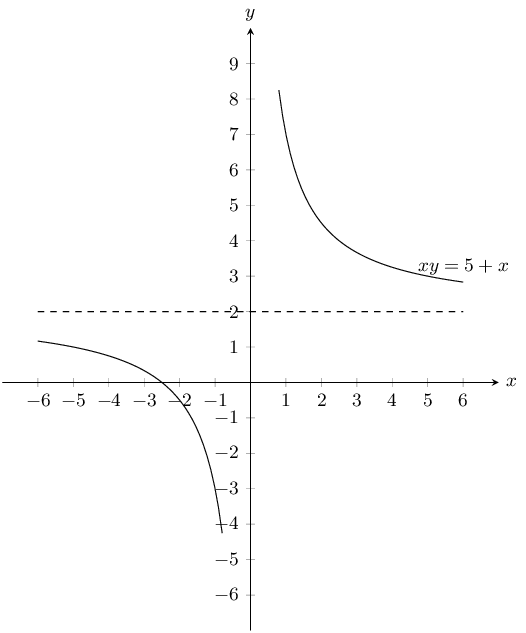$$2y + 2x = 3$$

First write the equation in standard form:

\begin{align*} 2y + 2x &= 3 \\ y &= -x + \frac{3}{2} \end{align*}